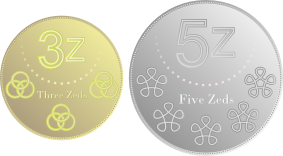#### You may also like### Where Can We Visit?

Charlie and Abi put a counter on 42. They wondered if they could visit all the other numbers on their 1-100 board, moving the counter using just these two operations: x2 and -5. What do you think?### Arithmagons

Can you find the values at the vertices when you know the values on the edges?### Babylon Numbers

Can you make a hypothesis to explain these ancient numbers?

# How Much Can We Spend?

##### Age 11 to 14Challenge Level

A country has decided to have just two different coins.

It has been suggested that these should be 3z and 5z coins.The shops think this is a good idea since most totals can be made.$2\times3z+1\times 5z=11z$ $7 \times 3z + 2 \times 5z = 31z$

Unfortunately some totals can't be made, for example 4z.

Is there a largest total that cannot be made?

How do you know?

They have decided that they will definitely have 3z coins but can't make up their minds about the other coin.

Experiment with other pairings containing 3z, and explore which totals can be made.

Can you find a relationship between 3z, the second coin, and the totals that can and can't be made?

In other countries they have also decided to have just two coins, but instead of the 3z coins they have chosen a different prime number.

Can you find a relationship between pairs of coin values and the totals that can and can't be made with them?

NOTES AND BACKGROUND

The coin problem (also referred to as Frobenius coin problem or Frobenius problem) is a mathematics problem associated with the German mathematician Ferdinand Georg Frobenius and often introduced in the context of making exact change given the availability of coins of specific denominations. To read about it go to Wikipedia.